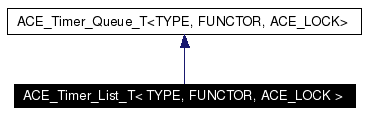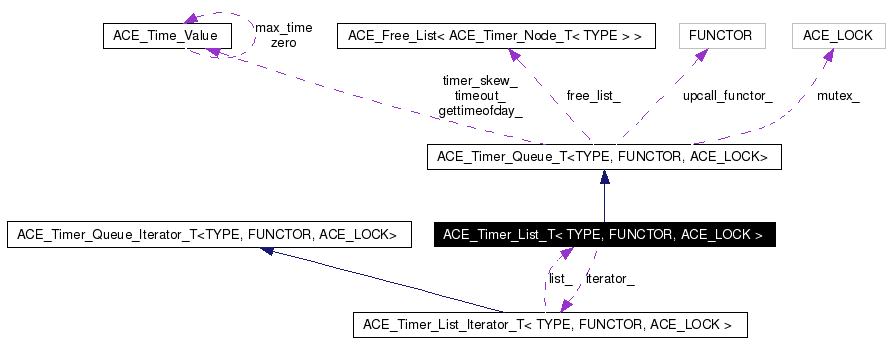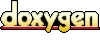# ACE_Timer_List_T< TYPE, FUNCTOR, ACE_LOCK > Class Template Reference

Provides a simple implementation of timers. More...

`#include <Timer_List_T.h>`

Inheritance diagram for ACE_Timer_List_T< TYPE, FUNCTOR, ACE_LOCK >:[legend]
Collaboration diagram for ACE_Timer_List_T< TYPE, FUNCTOR, ACE_LOCK >:[legend]
List of all members.

## Public Types

typedef ACE_Timer_List_Iterator_T<
TYPE, FUNCTOR, ACE_LOCK >
Iterator
Type of iterator.

typedef ACE_Timer_Node_T<
TYPE >
Node
typedef ACE_Timer_Queue_T<
TYPE, FUNCTOR, ACE_LOCK >
Base
Type inherited from.

typedef ACE_Free_List< NodeFreeList

## Public Member Functions

ACE_Timer_List_T (FUNCTOR *upcall_functor=0, FreeList *freelist=0)
virtual ~ACE_Timer_List_T (void)
Destructor.

virtual int is_empty (void) const
True if queue is empty, else false.

virtual const ACE_Time_Valueearliest_time (void) const
virtual int reset_interval (long timer_id, const ACE_Time_Value &interval)
virtual int cancel (const TYPE &type, int dont_call_handle_close=1)
virtual int cancel (long timer_id, const void **act=0, int dont_call_handle_close=1)
virtual ACE_Timer_Queue_Iterator_T<
TYPE, FUNCTOR, ACE_LOCK > &
iter (void)
Returns a pointer to this ACE_Timer_Queue's iterator.

virtual ACE_Timer_Node_T<
TYPE > *
remove_first (void)
Removes the earliest node from the queue and returns it.

virtual void dump (void) const
Dump the state of an object.

virtual void reschedule (ACE_Timer_Node_T< TYPE > *)
virtual ACE_Timer_Node_T<
TYPE > *
get_first (void)
Reads the earliest node from the queue and returns it.

## Private Member Functions

virtual long schedule_i (const TYPE &type, const void *act, const ACE_Time_Value &future_time, const ACE_Time_Value &interval)
void schedule_i (ACE_Timer_Node_T< TYPE > *n, const ACE_Time_Value &exp)
The shared scheduling functionality between schedule() and reschedule().

ACE_Timer_Node_T< TYPE > * find_node (long timer_id) const
void cancel_i (ACE_Timer_Node_T< TYPE > *n)
Shared subset of the two cancel() methods.

void unlink (ACE_Timer_Node_T< TYPE > *n)
ACE_Timer_Node_T< TYPE > * get_first_i (void) const
ACE_Timer_List_T (const ACE_Timer_List_T< TYPE, FUNCTOR, ACE_LOCK > &)
void operator= (const ACE_Timer_List_T< TYPE, FUNCTOR, ACE_LOCK > &)

## Private Attributes

ACE_Timer_Node_T< TYPE > * head_
Pointer to linked list of .

Iteratoriterator_
Iterator used to expire timers.

long id_counter_

## Friends

class ACE_Timer_List_Iterator_T< TYPE, FUNCTOR, ACE_LOCK >
Iterator is a friend.

## Detailed Description

### template<class TYPE, class FUNCTOR, class ACE_LOCK> class ACE_Timer_List_T< TYPE, FUNCTOR, ACE_LOCK >

Provides a simple implementation of timers.

This implementation uses a linked list of absolute times. Therefore, in the average case, scheduling and canceling timers is O(N) (where N is the total number of timers) and expiring timers is O(K) (where K is the total number of timers that are < the current time of day). More clever implementations could use a delta-list, a heap, or timing wheels, etc. For instance, ACE_Timer_Heap is a subclass of ACE_Timer_List that implements a heap-based callout queue. For most applications, the ACE_Timer_Heap will perform substantially faster than the ACE_Timer_List.

Definition at line 85 of file Timer_List_T.h.

## Member Typedef Documentation

 template typedef ACE_Timer_Queue_T ACE_Timer_List_T< TYPE, FUNCTOR, ACE_LOCK >::Base
 Type inherited from. Definition at line 96 of file Timer_List_T.h.

 template typedef ACE_Free_List ACE_Timer_List_T< TYPE, FUNCTOR, ACE_LOCK >::FreeList
 Definition at line 97 of file Timer_List_T.h.

 template typedef ACE_Timer_List_Iterator_T ACE_Timer_List_T< TYPE, FUNCTOR, ACE_LOCK >::Iterator
 Type of iterator. Definition at line 89 of file Timer_List_T.h.

 template typedef ACE_Timer_Node_T ACE_Timer_List_T< TYPE, FUNCTOR, ACE_LOCK >::Node
 Definition at line 94 of file Timer_List_T.h.

## Constructor & Destructor Documentation

 template ACE_Timer_List_T< TYPE, FUNCTOR, ACE_LOCK >::ACE_Timer_List_T ( FUNCTOR * upcall_functor = 0, FreeList * freelist = 0 )
 Default constructor. upcall_functor is the instance of the FUNCTOR to be used by the list. If upcall_functor is 0, a default FUNCTOR will be created. freelist is the freelist of timer nodes. If 0, then a default freelist will be created. Definition at line 83 of file Timer_List_T.cpp. ```00084 : Base(uf, fl) 00085 , head_ (new ACE_Timer_Node_T) 00086 , id_counter_ (0) 00087 { 00088 ACE_TRACE ("ACE_Timer_List_T::ACE_Timer_List_T"); 00089 00090 this->head_->set_next (this->head_); 00091 this->head_->set_prev (this->head_); 00092 00093 ACE_NEW (iterator_, Iterator(*this)); 00094 } ```

 template ACE_Timer_List_T< TYPE, FUNCTOR, ACE_LOCK >::~ACE_Timer_List_T ( void ) ` [virtual]`
 Destructor. Definition at line 123 of file Timer_List_T.cpp. ```00124 { 00125 ACE_TRACE ("ACE_Timer_List_T::~ACE_Timer_List_T"); 00126 ACE_MT (ACE_GUARD (ACE_LOCK, ace_mon, this->mutex_)); 00127 00128 delete iterator_; 00129 00130 if (!this->is_empty()) 00131 { 00132 for (ACE_Timer_Node_T* n = this->get_first(); 00133 n != this->head_; 00134 ) 00135 { 00136 this->upcall_functor ().deletion (*this, 00137 n->get_type(), 00138 n->get_act()); 00139 00140 ACE_Timer_Node_T *next = 00141 n->get_next (); 00142 00143 this->free_node (n); 00144 00145 n = next; 00146 } 00147 } 00148 00149 // delete the dummy node 00150 delete this->head_; 00151 } ```

 template ACE_Timer_List_T< TYPE, FUNCTOR, ACE_LOCK >::ACE_Timer_List_T ( const ACE_Timer_List_T< TYPE, FUNCTOR, ACE_LOCK > & ) ` [private]`

## Member Function Documentation

 template int ACE_Timer_List_T< TYPE, FUNCTOR, ACE_LOCK >::cancel ( long timer_id, const void ** act = 0, int dont_call_handle_close = 1 ) ` [virtual]`
 Cancel the single timer that matches the timer_id value (which was returned from the method). If act is non-NULL then it will be set to point to the ``magic cookie'' argument passed in when the timer was registered. This makes it possible to free up the memory and avoid memory leaks. If is 0 then the will be invoked. Returns 1 if cancellation succeeded and 0 if the wasn't found. Implements ACE_Timer_Queue_T< TYPE, FUNCTOR, ACE_LOCK >. Definition at line 277 of file Timer_List_T.cpp. ```00280 { 00281 ACE_TRACE ("ACE_Timer_List_T::cancel"); 00282 ACE_MT (ACE_GUARD_RETURN (ACE_LOCK, ace_mon, this->mutex_, -1)); 00283 ACE_Timer_Node_T* n = this->find_node(timer_id); 00284 if (n != 0) 00285 { 00286 if (act != 0) 00287 *act = n->get_act (); 00288 00289 // Call the close hooks. 00290 int cookie = 0; 00291 00292 // cancel_type() called once per . 00293 this->upcall_functor ().cancel_type (*this, 00294 n->get_type (), 00295 skip_close, 00296 cookie); 00297 00298 // cancel_timer() called once per . 00299 this->upcall_functor ().cancel_timer (*this, 00300 n->get_type (), 00301 skip_close, 00302 cookie); 00303 00304 this->cancel_i (n); 00305 00306 return 1; 00307 } 00308 00309 return 0; 00310 } ```

 template int ACE_Timer_List_T< TYPE, FUNCTOR, ACE_LOCK >::cancel ( const TYPE & type, int dont_call_handle_close = 1 ) ` [virtual]`
 Cancel all timers associated with type. If dont_call_handle_close is 0 then the functor will be invoked. Returns the number of timers cancelled. Implements ACE_Timer_Queue_T< TYPE, FUNCTOR, ACE_LOCK >. Definition at line 314 of file Timer_List_T.cpp. ```00315 { 00316 ACE_TRACE ("ACE_Timer_List_T::cancel"); 00317 ACE_MT (ACE_GUARD_RETURN (ACE_LOCK, ace_mon, this->mutex_, -1)); 00318 00319 int num_canceled = 0; // Note : Technically this can overflow. 00320 00321 if (!this->is_empty ()) 00322 { 00323 for (ACE_Timer_Node_T* n = this->get_first(); 00324 n != this->head_; 00325 ) 00326 { 00327 if (n->get_type() == type) // Note: Typically Type is an ACE_Event_Handler* 00328 { 00329 ++num_canceled; 00330 00331 ACE_Timer_Node_T* tmp = n; 00332 n = n->get_next(); 00333 00334 this->cancel_i (tmp); 00335 } 00336 else 00337 { 00338 n = n->get_next(); 00339 } 00340 } 00341 } 00342 00343 // Call the close hooks. 00344 int cookie = 0; 00345 00346 // cancel_type() called once per . 00347 this->upcall_functor ().cancel_type (*this, 00348 type, 00349 skip_close, 00350 cookie); 00351 00352 for (int i = 0; 00353 i < num_canceled; 00354 ++i) 00355 { 00356 // cancel_timer() called once per . 00357 this->upcall_functor ().cancel_timer (*this, 00358 type, 00359 skip_close, 00360 cookie); 00361 } 00362 00363 return num_canceled; 00364 } ```

 template void ACE_Timer_List_T< TYPE, FUNCTOR, ACE_LOCK >::cancel_i ( ACE_Timer_Node_T< TYPE > * n ) ` [private]`
 Shared subset of the two cancel() methods. Definition at line 377 of file Timer_List_T.cpp. Referenced by ACE_Timer_List_T< TYPE, FUNCTOR, ACE_LOCK >::cancel(). ```00378 { 00379 this->unlink (n); 00380 this->free_node (n); 00381 } ```

 template void ACE_Timer_List_T< TYPE, FUNCTOR, ACE_LOCK >::dump ( void ) const` [virtual]`
 Dump the state of an object. Reimplemented from ACE_Timer_Queue_T< TYPE, FUNCTOR, ACE_LOCK >. Definition at line 154 of file Timer_List_T.cpp. ```00155 { 00156 #if defined (ACE_HAS_DUMP) 00157 ACE_TRACE ("ACE_Timer_List_T::dump"); 00158 ACE_DEBUG ((LM_DEBUG, ACE_BEGIN_DUMP, this)); 00159 00160 int count = 0; 00161 00162 ACE_Timer_Node_T* n = this->get_first_i(); 00163 if (n != 0) { 00164 for (; n != this->head_; n = n->get_next()) { 00165 ++count; 00166 } 00167 } 00168 00169 ACE_DEBUG ((LM_DEBUG, ACE_LIB_TEXT ("\nsize_ = %d"), count)); 00170 ACE_DEBUG ((LM_DEBUG, ACE_END_DUMP)); 00171 #endif /* ACE_HAS_DUMP */ 00172 } ```

 template const ACE_Time_Value & ACE_Timer_List_T< TYPE, FUNCTOR, ACE_LOCK >::earliest_time ( void ) const` [virtual]`
 Returns the time of the earlier node in the ACE_Timer_List. Must be called on a non-empty queue. Implements ACE_Timer_Queue_T< TYPE, FUNCTOR, ACE_LOCK >. Definition at line 110 of file Timer_List_T.cpp. ```00111 { 00112 ACE_TRACE ("ACE_Timer_List_T::earliest_time"); 00113 ACE_Timer_Node_T* first = this->get_first_i(); 00114 if (first != 0) 00115 return first->get_timer_value (); 00116 return ACE_Time_Value::zero; 00117 } ```

 template ACE_Timer_Node_T< TYPE > * ACE_Timer_List_T< TYPE, FUNCTOR, ACE_LOCK >::find_node ( long timer_id ) const` [private]`
 Definition at line 245 of file Timer_List_T.cpp. ```00246 { 00247 ACE_Timer_Node_T* n = this->get_first_i(); 00248 if (n == 0) 00249 return 0; 00250 00251 for (; n != this->head_; n = n->get_next()) { 00252 if (n->get_timer_id() == timer_id) { 00253 return n; 00254 } 00255 } 00256 return 0; 00257 } ```

 template ACE_Timer_Node_T< TYPE > * ACE_Timer_List_T< TYPE, FUNCTOR, ACE_LOCK >::get_first ( void ) ` [virtual]`
 Reads the earliest node from the queue and returns it. Implements ACE_Timer_Queue_T< TYPE, FUNCTOR, ACE_LOCK >. Definition at line 385 of file Timer_List_T.cpp. ```00386 { 00387 ACE_TRACE ("ACE_Timer_List_T::get_first"); 00388 return this->get_first_i(); 00389 } ```

 template ACE_Timer_Node_T< TYPE > * ACE_Timer_List_T< TYPE, FUNCTOR, ACE_LOCK >::get_first_i ( void ) const` [private]`
 Definition at line 392 of file Timer_List_T.cpp. References ACE_TRACE. ```00393 { 00394 ACE_TRACE ("ACE_Timer_List_T::get_first_i"); 00395 ACE_Timer_Node_T* first = this->head_->get_next(); 00396 if (first != this->head_) // Note : is_empty() uses get_first() 00397 return first; 00398 return 0; 00399 } ```

 template int ACE_Timer_List_T< TYPE, FUNCTOR, ACE_LOCK >::is_empty ( void ) const` [virtual]`
 True if queue is empty, else false. Implements ACE_Timer_Queue_T< TYPE, FUNCTOR, ACE_LOCK >. Definition at line 100 of file Timer_List_T.cpp. ```00101 { 00102 ACE_TRACE ("ACE_Timer_List_T::is_empty"); 00103 return this->get_first_i() == 0; 00104 } ```

 template ACE_Timer_Queue_Iterator_T< TYPE, FUNCTOR, ACE_LOCK > & ACE_Timer_List_T< TYPE, FUNCTOR, ACE_LOCK >::iter ( void ) ` [virtual]`
 Returns a pointer to this ACE_Timer_Queue's iterator. Implements ACE_Timer_Queue_T< TYPE, FUNCTOR, ACE_LOCK >. Definition at line 74 of file Timer_List_T.cpp. ```00075 { 00076 this->iterator_->first (); 00077 return *this->iterator_; 00078 } ```

 template void ACE_Timer_List_T< TYPE, FUNCTOR, ACE_LOCK >::operator= ( const ACE_Timer_List_T< TYPE, FUNCTOR, ACE_LOCK > & ) ` [private]`

 template ACE_Timer_Node_T< TYPE > * ACE_Timer_List_T< TYPE, FUNCTOR, ACE_LOCK >::remove_first ( void ) ` [virtual]`
 Removes the earliest node from the queue and returns it. Implements ACE_Timer_Queue_T< TYPE, FUNCTOR, ACE_LOCK >. Definition at line 405 of file Timer_List_T.cpp. ```00406 { 00407 ACE_TRACE ("ACE_Timer_List_T::remove_first"); 00408 ACE_Timer_Node_T* first = this->get_first(); 00409 if (first != 0) { 00410 this->unlink(first); 00411 return first; 00412 } 00413 return 0; 00414 } ```

 template void ACE_Timer_List_T< TYPE, FUNCTOR, ACE_LOCK >::reschedule ( ACE_Timer_Node_T< TYPE > * ) ` [virtual]`
 Reschedule an "interval" ACE_Timer_Node_T. This should be private but for now it needs to be public for Implements ACE_Timer_Queue_T< TYPE, FUNCTOR, ACE_LOCK >. Definition at line 179 of file Timer_List_T.cpp. ```00180 { 00181 ACE_TRACE ("ACE_Timer_List_T::reschedule"); 00182 this->schedule_i(n, n->get_timer_value()); 00183 } ```

 template int ACE_Timer_List_T< TYPE, FUNCTOR, ACE_LOCK >::reset_interval ( long timer_id, const ACE_Time_Value & interval ) ` [virtual]`
 Resets the interval of the timer represented by timer_id to interval, which is specified in relative time to the current . If interval is equal to ACE_Time_Value::zero, the timer will become a non-rescheduling timer. Returns 0 if successful, -1 if not. Implements ACE_Timer_Queue_T< TYPE, FUNCTOR, ACE_LOCK >. Definition at line 261 of file Timer_List_T.cpp. ```00263 { 00264 ACE_TRACE ("ACE_Timer_List_T::reset_interval"); 00265 ACE_MT (ACE_GUARD_RETURN (ACE_LOCK, ace_mon, this->mutex_, -1)); 00266 ACE_Timer_Node_T* n = this->find_node(timer_id); 00267 if (n != 0) { 00268 n->set_interval(interval); // The interval will take effect the next time this node is expired. 00269 return 0; 00270 } 00271 return -1; 00272 } ```

 template void ACE_Timer_List_T< TYPE, FUNCTOR, ACE_LOCK >::schedule_i ( ACE_Timer_Node_T< TYPE > * n, const ACE_Time_Value & exp ) ` [private]`
 The shared scheduling functionality between schedule() and reschedule(). Definition at line 217 of file Timer_List_T.cpp. ```00219 { 00220 if (this->is_empty()) { 00221 n->set_prev(this->head_); 00222 n->set_next(this->head_); 00223 this->head_->set_prev(n); 00224 this->head_->set_next(n); 00225 return; 00226 } 00227 00228 // We always want to search backwards from the tail of the list, because 00229 // this minimizes the search in the extreme case when lots of timers are 00230 // scheduled for exactly the same time, and it also assumes that most of 00231 // the timers will be scheduled later than existing timers. 00232 ACE_Timer_Node_T* p = this->head_->get_prev(); 00233 while (p != this->head_ && p->get_timer_value() > expire) 00234 p = p->get_prev(); 00235 00236 // insert after 00237 n->set_prev(p); 00238 n->set_next(p->get_next()); 00239 p->get_next()->set_prev(n); 00240 p->set_next(n); 00241 } ```

 template long ACE_Timer_List_T< TYPE, FUNCTOR, ACE_LOCK >::schedule_i ( const TYPE & type, const void * act, const ACE_Time_Value & future_time, const ACE_Time_Value & interval ) ` [private, virtual]`
 Schedule that will expire at , which is specified in absolute time. If it expires then is passed in as the value to the . If is != to then it is used to reschedule the automatically, using relative time to the current . This method returns a that uniquely identifies the the entry in an internal list. This can be used to cancel the timer before it expires. The cancellation ensures that are unique up to values of greater than 2 billion timers. As long as timers don't stay around longer than this there should be no problems with accidentally deleting the wrong timer. Returns -1 on failure (which is guaranteed never to be a valid ). Implements ACE_Timer_Queue_T< TYPE, FUNCTOR, ACE_LOCK >. Definition at line 190 of file Timer_List_T.cpp. ```00194 { 00195 ACE_TRACE ("ACE_Timer_List_T::schedule_i"); 00196 00197 ACE_Timer_Node_T* n = this->alloc_node(); 00198 00199 if (n != 0) 00200 { 00201 long id = this->id_counter_++; 00202 00203 if (id != -1) { 00204 n->set (type, act, future_time, interval, 0, 0, id); 00205 this->schedule_i (n, future_time); 00206 } 00207 return id; 00208 } 00209 00210 // Failure return 00211 errno = ENOMEM; 00212 return -1; 00213 } ```

 template void ACE_Timer_List_T< TYPE, FUNCTOR, ACE_LOCK >::unlink ( ACE_Timer_Node_T< TYPE > * n ) ` [private]`
 Definition at line 367 of file Timer_List_T.cpp. ```00368 { 00369 n->get_prev()->set_next(n->get_next()); 00370 n->get_next()->set_prev(n->get_prev()); 00371 n->set_prev(0); 00372 n->set_next(0); 00373 } ```

## Friends And Related Function Documentation

 template friend class ACE_Timer_List_Iterator_T< TYPE, FUNCTOR, ACE_LOCK >` [friend]`
 Iterator is a friend. Definition at line 92 of file Timer_List_T.h.

## Member Data Documentation

 template ACE_Timer_Node_T* ACE_Timer_List_T< TYPE, FUNCTOR, ACE_LOCK >::head_` [private]`
 Pointer to linked list of . Definition at line 200 of file Timer_List_T.h.

 template long ACE_Timer_List_T< TYPE, FUNCTOR, ACE_LOCK >::id_counter_` [private]`
 Keeps track of the timer id that uniquely identifies each timer. This id can be used to cancel a timer via the method. Definition at line 210 of file Timer_List_T.h.

 template Iterator* ACE_Timer_List_T< TYPE, FUNCTOR, ACE_LOCK >::iterator_` [private]`
 Iterator used to expire timers. Definition at line 203 of file Timer_List_T.h.

The documentation for this class was generated from the following files:
Generated on Thu Nov 9 11:31:20 2006 for ACE by1.3.6